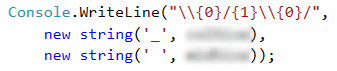# Problem: Drawing a Fort

Write a program that reads from the console an integer n and draws a fort 2 * n columns wide and n rows tall, as in the examples below. The left and right columns inside are n / 2 wide.

## Input Data

The input is an integer n within the range [3 … 1000].

## Output Data

Print on the console n text rows, depicting the fort exactly as in the examples.

## Sample Input and Output

Input Output Input Output
3 /^\/^\
|    |
\_/\_/
4 /^^\/^^\
|      |
|      |
\__/\__/
Input Output Input Output
5 /^^\__/^^\
|        |
|        |
|   __   |
\__/  \__/
8 /^^^^\____/^^^^\
|              |
|              |
|              |
|              |
|              |
|     ____     |
\____/    \____/

## Hints and Guidelines

Let’s solve the problem step by step: read the input, perform some calculations, print the fort roof, print the fort body, print the fort base.

We can see from the explanation that the input data will be only one line which will contain an integer within the range [3 … 1000]. Therefore, we will use a variable of int type.After we have declared and initialized the input data, we must divide the fort into three parts:

• roof
• body
• base

### Calculating and Printing the Roof

We can see from the examples that the roof is made of two towers and a middle part. Each tower has a beginning /, middle part ^ and an end \.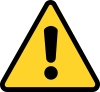\ is a special symbol in C# and using only it in the method Console.WriteLine(…), the console will not print it, that's why we show with \\ that we want to print exactly this symbol, without being interpreted as a special symbol (this is called "character escaping").

The size of the middle part is n / 2, therefore we can write this value in a new variable. It will keep the size of the middle part of the tower.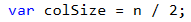Now we declare a second variable, in which will keep the value of the part between the two towers. The middle part of the roofs has a size of 2 * n - 2 * colSize - 4.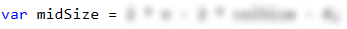In order to print the roof, we will use new string, which takes two parameters (char, int) and connects a symbol n times.### Printing the Body of the Fort

The body of the fort contains a beginning |, a middle part (white space) and an end |. The middle part is a blank space with size of 2 * n - 2. The number of the rows used for walls can be found in the given parameters: n - 3. This code prints the body of the fort:### Printing the Base of the Fort

In order to draw the last but one row, which is a part of the base, we need to print a beginning |, middle part (white space)_(white space) and an end |. In order to do this, we can use the already declared variables colSize and midSize again, because we can see from the examples that they are equal to the _ in the roof.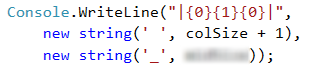We add + 1 to the white spaces, because we have one white space more in the examples.

The structure of the base of the fort is the same as the one of the roof. It is made of two towers and a middle part. Each tower begins with \, then a middle part _ and an end /.